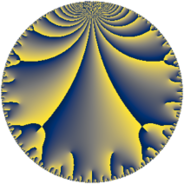# Properties

 Label 91.8.rLevel $91$ Weight $8$ Character orbit 91.r Rep. character $\chi_{91}(25,\cdot)$ Character field $\Q(\zeta_{6})$ Dimension $128$ Newform subspaces $1$ Sturm bound $74$ Trace bound $0$

# Related objects

## Defining parameters

 Level: $$N$$ $$=$$ $$91 = 7 \cdot 13$$ Weight: $$k$$ $$=$$ $$8$$ Character orbit: $$[\chi]$$ $$=$$ 91.r (of order $$6$$ and degree $$2$$) Character conductor: $$\operatorname{cond}(\chi)$$ $$=$$ $$91$$ Character field: $$\Q(\zeta_{6})$$ Newform subspaces: $$1$$ Sturm bound: $$74$$ Trace bound: $$0$$

## Dimensions

The following table gives the dimensions of various subspaces of $$M_{8}(91, [\chi])$$.

Total New Old
Modular forms 136 136 0
Cusp forms 128 128 0
Eisenstein series 8 8 0

## Trace form

 $$128 q + 52 q^{3} + 4094 q^{4} - 44052 q^{9} + O(q^{10})$$ $$128 q + 52 q^{3} + 4094 q^{4} - 44052 q^{9} - 4514 q^{10} + 2090 q^{12} + 11132 q^{13} + 18748 q^{14} - 230074 q^{16} - 28972 q^{17} + 179920 q^{22} + 20508 q^{23} + 920040 q^{25} + 144684 q^{26} - 357584 q^{27} + 44600 q^{29} + 46580 q^{30} - 1216824 q^{35} - 4529248 q^{36} - 129032 q^{38} + 104546 q^{39} + 2082020 q^{40} + 2426496 q^{42} - 1434416 q^{43} - 3783940 q^{48} - 34428 q^{49} - 756008 q^{51} - 2348612 q^{52} + 1103824 q^{53} - 4216216 q^{55} + 8135574 q^{56} + 531568 q^{61} + 26627120 q^{62} - 27402496 q^{64} - 7495542 q^{65} + 2808402 q^{66} + 531760 q^{68} + 24238760 q^{69} - 7462590 q^{74} - 1547944 q^{75} - 1811116 q^{77} - 31233508 q^{78} + 2961020 q^{79} - 34639872 q^{81} - 10292872 q^{82} + 43455484 q^{87} + 21936790 q^{88} - 58442348 q^{90} + 16479848 q^{91} + 24834900 q^{92} + 26036992 q^{94} + 89048 q^{95} + O(q^{100})$$

## Decomposition of $$S_{8}^{\mathrm{new}}(91, [\chi])$$ into newform subspaces

Label Dim $A$ Field CM Traces $q$-expansion
$a_{2}$ $a_{3}$ $a_{5}$ $a_{7}$
91.8.r.a $128$ $28.427$ None $$0$$ $$52$$ $$0$$ $$0$$